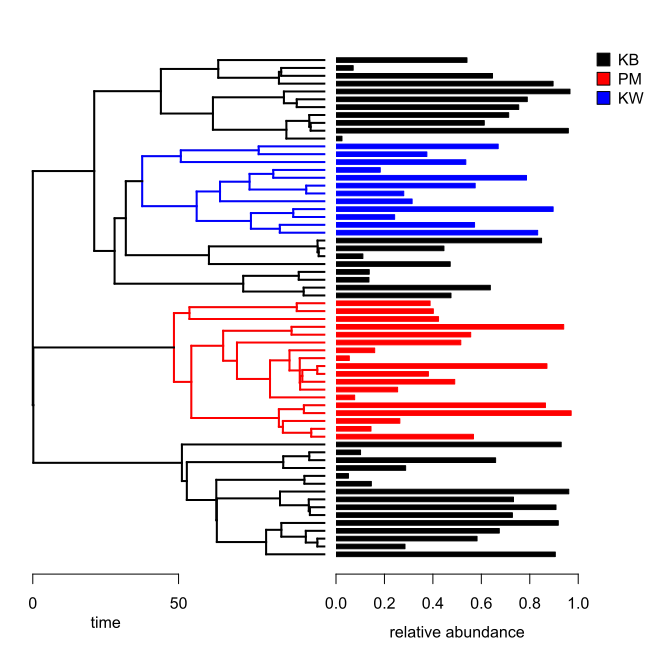## Thursday, May 17, 2018

### Customizing a bar plot paired with a plotted phylogeny

Yesterday a phytools user posted the following comment about the function `plotTree.barplot`:

“Very neat tool to plot barchart next to a tree. Is there a way to color the branches, and also add an axis and axis title to the tree?

Using the following code did nothing to add color the the branch:

``````for(i in 1:length(tt)) tree<-paintSubTree(tree,node=i,state=tt[i],stem=TRUE)
colors<-setNames(c("black","red","blue"),c("KB","PM","KW"))
ftype="reg", colors=colors,lwd=4, use.edge.length = TRUE,axis(1)),
args.barplot=list(xlab="Relative abundance",col=phylum_colors, xlim=c(0,1),
legend.text=TRUE,args.legend = list(x = "topright", bty="n", xpd = TRUE)))
``````

There is also no free space to plot the legend. Any help is much appreciated.”

Since the commenter hasn't sent me the original data I had to invent some. Here is what they look like:

``````library(phytools)
tree
``````
``````##
## Phylogenetic tree with 64 tips and 63 internal nodes.
##
## Tip labels:
##  t10, t60, t61, t45, t20, t52, ...
##
## Rooted; includes branch lengths.
``````
``````x
``````
``````##        t10        t60        t61        t45        t20        t52
## 0.90532233 0.28482390 0.58228935 0.67411616 0.91762887 0.72831160
##        t53        t48        t17        t43        t44        t22
## 0.90828956 0.73272700 0.96101692 0.14584122 0.05139047 0.28754064
##        t50        t51         t2        t56        t57        t21
## 0.65900185 0.10136811 0.92964094 0.56752999 0.14451551 0.26363247
##        t41        t42        t11        t37        t38        t58
## 0.97087520 0.86466752 0.07710963 0.25440640 0.49022645 0.38157265
##        t59        t36        t25         t7        t26        t27
## 0.87092899 0.05497484 0.15975640 0.51522823 0.55614193 0.94013214
##         t3        t32        t33        t39        t40        t34
## 0.42338521 0.40184781 0.38844121 0.47471898 0.63702061 0.13603071
##        t35         t4        t63        t64        t62        t15
## 0.13721665 0.47143131 0.11097818 0.44565765 0.84875954 0.83262279
##        t16        t28        t29         t6        t46        t47
## 0.57204090 0.24215227 0.89643177 0.31423521 0.27973032 0.57452690
##        t12        t13         t1         t8         t9        t24
## 0.78650892 0.18264281 0.53596682 0.37515409 0.66952881 0.02464721
##        t54        t55        t49        t30        t31        t23
## 0.95914836 0.61207190 0.71294626 0.75437136 0.78962420 0.96600007
##        t14        t18        t19         t5
## 0.89622112 0.64615452 0.07087410 0.54074719
``````

Now, this inquiry had many parts. If I have them right, he or she wants to:

1) Add a axis & axis title to the tree. 2) Leave space on the barplot for a legend & add it. 3) Color the edges of the tree by painting subtrees different colors.

All of this is possible - though not necessarily in the way the commenter imagines.

In addition, I had to push a couple of small updates to `plotTree.barplot` to get the following to work exactly as hoped. These can be obtained simply by updating phytools from GitHub. If you don't want to do that this second you can instead load the function from source as follows (although I recommend updating phytools the next time you restart R):

``````source("https://raw.githubusercontent.com/liamrevell/phytools/master/R/plotTree.wBars.R")
``````

Now we're ready! Let's see it:

``````## first paint sub trees
tree<-paintSubTree(tree,81,"PM","KB")
tree<-paintSubTree(tree,107,"KW","KB")
## set colors
colors<-setNames(c("black","red","blue"),c("KB","PM","KW"))
``````

Next I'm going to pull the 'states' (based on my above painting) from the tips. This is just to color the bars using the same scheme as I've used for the edges of the tree.

``````ss<-getStates(tree,"tips")
barcols<-setNames(sapply(ss,function(x,y) y[which(names(y)==x)],
y=colors),names(ss))
``````

Now I'm ready to plot. Note that my initial plot is of a plain tree to the level panel - but then I will replot the tree in that space using the same parameters.

``````plotTree.barplot(tree,x[tree\$tip.label],args.plotTree=list(ftype="off"),
args.barplot=list(col=barcols,border=barcols,xlab="",
xlim=c(0,1.3)),args.axis=list(at=seq(0,1,by=0.2)))
legend(x="topright",legend=names(colors),pch=22,pt.cex=2,pt.bg=colors,
box.col="transparent")
mtext("relative abundance",1,at=0.5,line=2.5)
## this is how I go back to my tree frame
par(mfg=c(1,1))
plot(tree,colors,ftype='off',mar=c(5.1,1.1,2.1,0))
axis(1,at=seq(0,50,by=50))
mtext("time",1,at=25,line=2)
``````Neat. That's basically what I wanted.

FYI, the following shows how the tree & data were simulated for this demo:

``````set.seed(10)
tree<-pbtree(n=64,scale=100)
x<-fastBM(tree,sig2=0.1,bounds=c(0,1))
``````

1.Hi Liam,

I would like to change the tip.labels colours on a phylogeny based on a discrete trait value in a traits data.frame.

What is a method to do this? The above is a step too far for me at this stage.
Thanks a lot,
Jarrah

2.Thank you for providing cool examples for what we can do with this package! I have a non-ultrametric tree and I would like to plot next to a bar chart. I can't seem to both align tip labels (with dotted lines) and get the barplot to show. Is there a method for this?

Note: due to the very large amount of spam, all comments are now automatically submitted for moderation.